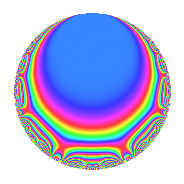Properties

 Label 4020.2.q.iLevel 4020 Weight 2 Character orbit 4020.q Analytic conductor 32.100 Analytic rank 0 Dimension 4 CM No Inner twists 2

Related objects

Newspace parameters

 Level: $$N$$ = $$4020 = 2^{2} \cdot 3 \cdot 5 \cdot 67$$ Weight: $$k$$ = $$2$$ Character orbit: $$[\chi]$$ = 4020.q (of order $$3$$ and degree $$2$$)

Newform invariants

 Self dual: No Analytic conductor: $$32.0998616126$$ Analytic rank: $$0$$ Dimension: $$4$$ Relative dimension: $$2$$ over $$\Q(\zeta_{3})$$ Coefficient field: $$\Q(\sqrt{-3}, \sqrt{-11})$$ Coefficient ring: $$\Z[a_1, \ldots, a_{13}]$$ Coefficient ring index: $$3$$ Sato-Tate group: $\mathrm{SU}(2)[C_{3}]$

$q$-expansion

Coefficients of the $$q$$-expansion are expressed in terms of a basis $$1,\beta_1,\beta_2,\beta_3$$ for the coefficient ring described below. We also show the integral $$q$$-expansion of the trace form.

 $$f(q)$$ $$=$$ $$q$$ $$+ q^{3}$$ $$- q^{5}$$ $$+ ( - \beta_{1} + \beta_{3} ) q^{7}$$ $$+ q^{9}$$ $$+O(q^{10})$$ $$q$$ $$+ q^{3}$$ $$- q^{5}$$ $$+ ( - \beta_{1} + \beta_{3} ) q^{7}$$ $$+ q^{9}$$ $$+ ( \beta_{1} + \beta_{3} ) q^{11}$$ $$+ ( 1 - \beta_{1} ) q^{13}$$ $$- q^{15}$$ $$+ ( -4 + 5 \beta_{1} + \beta_{2} - \beta_{3} ) q^{17}$$ $$+ ( -4 + 3 \beta_{1} - \beta_{2} + \beta_{3} ) q^{19}$$ $$+ ( - \beta_{1} + \beta_{3} ) q^{21}$$ $$+ ( -4 + 5 \beta_{1} + \beta_{2} - \beta_{3} ) q^{23}$$ $$+ q^{25}$$ $$+ q^{27}$$ $$+ ( - \beta_{1} - \beta_{3} ) q^{29}$$ $$+ ( - \beta_{1} - 2 \beta_{3} ) q^{31}$$ $$+ ( \beta_{1} + \beta_{3} ) q^{33}$$ $$+ ( \beta_{1} - \beta_{3} ) q^{35}$$ $$+ ( -2 - \beta_{1} - 3 \beta_{2} + 3 \beta_{3} ) q^{37}$$ $$+ ( 1 - \beta_{1} ) q^{39}$$ $$+ ( 7 \beta_{1} + \beta_{3} ) q^{41}$$ $$+ ( 1 + \beta_{2} ) q^{43}$$ $$- q^{45}$$ $$+ ( 5 \beta_{1} - \beta_{3} ) q^{47}$$ $$+ ( -1 + 2 \beta_{1} + \beta_{2} - \beta_{3} ) q^{49}$$ $$+ ( -4 + 5 \beta_{1} + \beta_{2} - \beta_{3} ) q^{51}$$ $$+ ( -4 - 2 \beta_{2} ) q^{53}$$ $$+ ( - \beta_{1} - \beta_{3} ) q^{55}$$ $$+ ( -4 + 3 \beta_{1} - \beta_{2} + \beta_{3} ) q^{57}$$ $$+ ( 2 - 2 \beta_{2} ) q^{59}$$ $$+ ( -11 + 11 \beta_{1} ) q^{61}$$ $$+ ( - \beta_{1} + \beta_{3} ) q^{63}$$ $$+ ( -1 + \beta_{1} ) q^{65}$$ $$+ ( 6 - 6 \beta_{1} - \beta_{2} - \beta_{3} ) q^{67}$$ $$+ ( -4 + 5 \beta_{1} + \beta_{2} - \beta_{3} ) q^{69}$$ $$+ ( -3 \beta_{1} - 3 \beta_{3} ) q^{71}$$ $$+ ( 13 - 13 \beta_{1} ) q^{73}$$ $$+ q^{75}$$ $$+ ( -8 + 7 \beta_{1} - \beta_{2} + \beta_{3} ) q^{77}$$ $$+ ( 11 \beta_{1} - 2 \beta_{3} ) q^{79}$$ $$+ q^{81}$$ $$+ ( -3 \beta_{1} - 3 \beta_{2} + 3 \beta_{3} ) q^{83}$$ $$+ ( 4 - 5 \beta_{1} - \beta_{2} + \beta_{3} ) q^{85}$$ $$+ ( - \beta_{1} - \beta_{3} ) q^{87}$$ $$+ ( -2 - 4 \beta_{2} ) q^{89}$$ $$+ \beta_{2} q^{91}$$ $$+ ( - \beta_{1} - 2 \beta_{3} ) q^{93}$$ $$+ ( 4 - 3 \beta_{1} + \beta_{2} - \beta_{3} ) q^{95}$$ $$+ ( 1 - \beta_{1} ) q^{97}$$ $$+ ( \beta_{1} + \beta_{3} ) q^{99}$$ $$+O(q^{100})$$ $$\operatorname{Tr}(f)(q)$$ $$=$$ $$4q$$ $$\mathstrut +\mathstrut 4q^{3}$$ $$\mathstrut -\mathstrut 4q^{5}$$ $$\mathstrut -\mathstrut q^{7}$$ $$\mathstrut +\mathstrut 4q^{9}$$ $$\mathstrut +\mathstrut O(q^{10})$$ $$4q$$ $$\mathstrut +\mathstrut 4q^{3}$$ $$\mathstrut -\mathstrut 4q^{5}$$ $$\mathstrut -\mathstrut q^{7}$$ $$\mathstrut +\mathstrut 4q^{9}$$ $$\mathstrut +\mathstrut 3q^{11}$$ $$\mathstrut +\mathstrut 2q^{13}$$ $$\mathstrut -\mathstrut 4q^{15}$$ $$\mathstrut -\mathstrut 9q^{17}$$ $$\mathstrut -\mathstrut 7q^{19}$$ $$\mathstrut -\mathstrut q^{21}$$ $$\mathstrut -\mathstrut 9q^{23}$$ $$\mathstrut +\mathstrut 4q^{25}$$ $$\mathstrut +\mathstrut 4q^{27}$$ $$\mathstrut -\mathstrut 3q^{29}$$ $$\mathstrut -\mathstrut 4q^{31}$$ $$\mathstrut +\mathstrut 3q^{33}$$ $$\mathstrut +\mathstrut q^{35}$$ $$\mathstrut -\mathstrut q^{37}$$ $$\mathstrut +\mathstrut 2q^{39}$$ $$\mathstrut +\mathstrut 15q^{41}$$ $$\mathstrut +\mathstrut 2q^{43}$$ $$\mathstrut -\mathstrut 4q^{45}$$ $$\mathstrut +\mathstrut 9q^{47}$$ $$\mathstrut -\mathstrut 3q^{49}$$ $$\mathstrut -\mathstrut 9q^{51}$$ $$\mathstrut -\mathstrut 12q^{53}$$ $$\mathstrut -\mathstrut 3q^{55}$$ $$\mathstrut -\mathstrut 7q^{57}$$ $$\mathstrut +\mathstrut 12q^{59}$$ $$\mathstrut -\mathstrut 22q^{61}$$ $$\mathstrut -\mathstrut q^{63}$$ $$\mathstrut -\mathstrut 2q^{65}$$ $$\mathstrut +\mathstrut 13q^{67}$$ $$\mathstrut -\mathstrut 9q^{69}$$ $$\mathstrut -\mathstrut 9q^{71}$$ $$\mathstrut +\mathstrut 26q^{73}$$ $$\mathstrut +\mathstrut 4q^{75}$$ $$\mathstrut -\mathstrut 15q^{77}$$ $$\mathstrut +\mathstrut 20q^{79}$$ $$\mathstrut +\mathstrut 4q^{81}$$ $$\mathstrut +\mathstrut 3q^{83}$$ $$\mathstrut +\mathstrut 9q^{85}$$ $$\mathstrut -\mathstrut 3q^{87}$$ $$\mathstrut -\mathstrut 2q^{91}$$ $$\mathstrut -\mathstrut 4q^{93}$$ $$\mathstrut +\mathstrut 7q^{95}$$ $$\mathstrut +\mathstrut 2q^{97}$$ $$\mathstrut +\mathstrut 3q^{99}$$ $$\mathstrut +\mathstrut O(q^{100})$$

Basis of coefficient ring in terms of a root $$\nu$$ of $$x^{4}\mathstrut -\mathstrut$$ $$x^{3}\mathstrut -\mathstrut$$ $$2$$ $$x^{2}\mathstrut -\mathstrut$$ $$3$$ $$x\mathstrut +\mathstrut$$ $$9$$:

 $$\beta_{0}$$ $$=$$ $$1$$ $$\beta_{1}$$ $$=$$ $$($$$$\nu^{3} + 2 \nu^{2} - 2 \nu - 3$$$$)/6$$ $$\beta_{2}$$ $$=$$ $$($$$$-\nu^{3} + \nu^{2} + 5 \nu$$$$)/3$$ $$\beta_{3}$$ $$=$$ $$($$$$2 \nu^{3} + \nu^{2} + 2 \nu - 9$$$$)/3$$
 $$1$$ $$=$$ $$\beta_0$$ $$\nu$$ $$=$$ $$($$$$\beta_{3}\mathstrut +\mathstrut$$ $$\beta_{2}\mathstrut -\mathstrut$$ $$2$$ $$\beta_{1}\mathstrut +\mathstrut$$ $$2$$$$)/3$$ $$\nu^{2}$$ $$=$$ $$($$$$-$$$$\beta_{3}\mathstrut +\mathstrut$$ $$2$$ $$\beta_{2}\mathstrut +\mathstrut$$ $$8$$ $$\beta_{1}\mathstrut +\mathstrut$$ $$1$$$$)/3$$ $$\nu^{3}$$ $$=$$ $$($$$$4$$ $$\beta_{3}\mathstrut -\mathstrut$$ $$2$$ $$\beta_{2}\mathstrut -\mathstrut$$ $$2$$ $$\beta_{1}\mathstrut +\mathstrut$$ $$11$$$$)/3$$

Character Values

We give the values of $$\chi$$ on generators for $$\left(\mathbb{Z}/4020\mathbb{Z}\right)^\times$$.

 $$n$$ $$1141$$ $$2011$$ $$2681$$ $$3217$$ $$\chi(n)$$ $$- \beta_{1}$$ $$1$$ $$1$$ $$1$$

Embeddings

For each embedding $$\iota_m$$ of the coefficient field, the values $$\iota_m(a_n)$$ are shown below.

For more information on an embedded modular form you can click on its label.

Label $$\iota_m(\nu)$$ $$a_{2}$$ $$a_{3}$$ $$a_{4}$$ $$a_{5}$$ $$a_{6}$$ $$a_{7}$$ $$a_{8}$$ $$a_{9}$$ $$a_{10}$$
841.1
 −1.18614 + 1.26217i 1.68614 − 0.396143i −1.18614 − 1.26217i 1.68614 + 0.396143i
0 1.00000 0 −1.00000 0 −1.68614 + 2.92048i 0 1.00000 0
841.2 0 1.00000 0 −1.00000 0 1.18614 2.05446i 0 1.00000 0
3781.1 0 1.00000 0 −1.00000 0 −1.68614 2.92048i 0 1.00000 0
3781.2 0 1.00000 0 −1.00000 0 1.18614 + 2.05446i 0 1.00000 0
 $$n$$: e.g. 2-40 or 990-1000 Significant digits: Format: Complex embeddings Normalized embeddings Satake parameters Satake angles

Inner twists

Char. orbit Parity Mult. Self Twist Proved
1.a Even 1 trivial yes
67.c Even 1 no

Hecke kernels

This newform can be constructed as the intersection of the kernels of the following linear operators acting on $$S_{2}^{\mathrm{new}}(4020, \chi)$$:

 $$T_{7}^{4}$$ $$\mathstrut +\mathstrut T_{7}^{3}$$ $$\mathstrut +\mathstrut 9 T_{7}^{2}$$ $$\mathstrut -\mathstrut 8 T_{7}$$ $$\mathstrut +\mathstrut 64$$ $$T_{11}^{4}$$ $$\mathstrut -\mathstrut 3 T_{11}^{3}$$ $$\mathstrut +\mathstrut 15 T_{11}^{2}$$ $$\mathstrut +\mathstrut 18 T_{11}$$ $$\mathstrut +\mathstrut 36$$ $$T_{17}^{4}$$ $$\mathstrut +\mathstrut 9 T_{17}^{3}$$ $$\mathstrut +\mathstrut 69 T_{17}^{2}$$ $$\mathstrut +\mathstrut 108 T_{17}$$ $$\mathstrut +\mathstrut 144$$